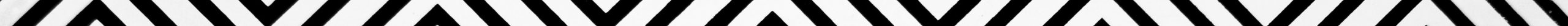## Equations

Absolute value

Linear equations in one variable

## Exponents

What are exponents?

Laws of Exponents

Completing the square

## Functions

Types of functions

## Inequalities

Absolute value

Absolute value inequalities

## Logarithms and Exponentials

Introduction to logarithms

Logarithms formula

Logarithm rules

## Polynomials

What are polynomials

How to divide polynomials.

## Probability

Fundamental rule of counting

Factorial of a number, n

Permutations and combinations

## Sequences and Series

Arithmetic sequence

Geometric sequence

## Set Theory

Set theory conceptsInsert math as
$${}$$